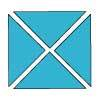#### You may also like### Chocolate

There are three tables in a room with blocks of chocolate on each. Where would be the best place for each child in the class to sit if they came in one at a time?### Four Triangles Puzzle

Cut four triangles from a square as shown in the picture. How many different shapes can you make by fitting the four triangles back together?### Cut it Out

Can you dissect an equilateral triangle into 6 smaller ones? What number of smaller equilateral triangles is it NOT possible to dissect a larger equilateral triangle into?

# Four-digit Targets

##### Age 7 to 11Challenge Level

Try using cards numbered from $0$ to $9$ or even pieces of torn paper.
Where is the best place to put $9$ when you are aiming for the lowest number?
Where is the best place to put $9$ when you are aiming for the highest number?